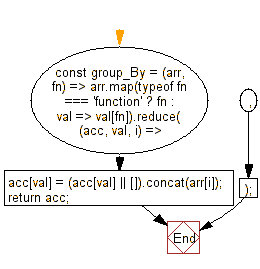# JavaScript: Group the elements of a given array based on the given function

## JavaScript fundamental (ES6 Syntax): Exercise-52 with Solution

Write a JavaScript program to group the elements of a given array based on the given function.

• Use Array.prototype.map() to map the values of the array to a function or property name.
• Use Array.prototype.reduce() to create an object, where the keys are produced from the mapped results.

Sample Solution:

JavaScript Code:

``````//#Source https://bit.ly/2neWfJ2
const group_By = (arr, fn) =>
arr.map(typeof fn === 'function' ? fn : val => val[fn]).reduce((acc, val, i) => {
acc[val] = (acc[val] || []).concat(arr[i]);
return acc;
}, {});
console.log(group_By([6.1, 4.2, 6.3], Math.sqrt));
console.log(group_By([6.1, 4.2, 6.3], Math.floor));
console.log(group_By(['one', 'two', 'three'], 'length'));
```
```

Sample Output:

```{"2.4698178070456938":[6.1],"2.04939015319192":[4.2],"2.5099800796022267":[6.3]}
{"4":[4.2],"6":[6.1,6.3]}
{"3":["one","two"],"5":["three"]}
```

Flowchart:Live Demo:

See the Pen javascript-basic-exercise-1-52 by w3resource (@w3resource) on CodePen.

Improve this sample solution and post your code through Disqus

What is the difficulty level of this exercise?

Test your Programming skills with w3resource's quiz.

﻿